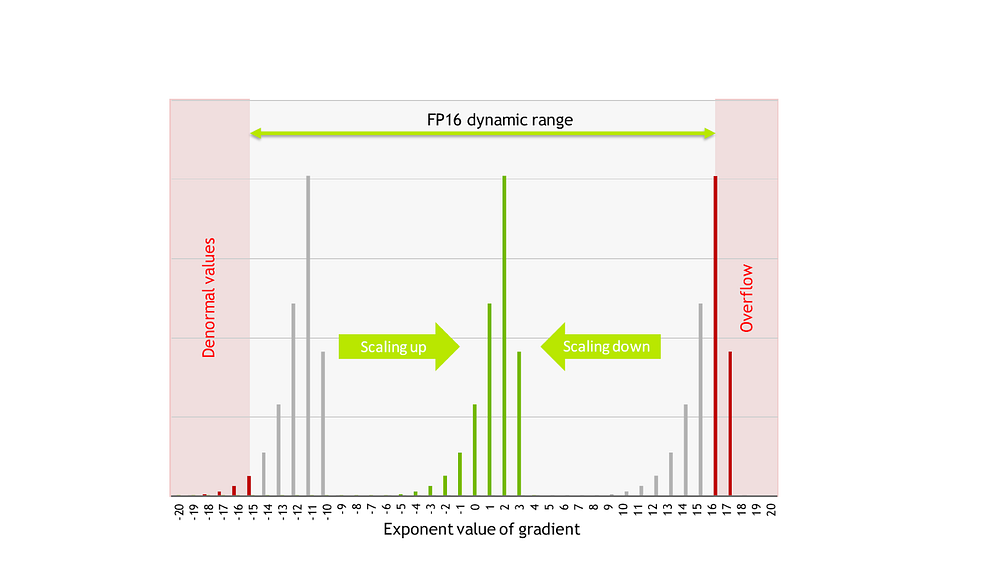# Simplify mixed precision training with MXNet-AMP

Source: Deep Learning on Medium# AMP in MXNet

A typical training loop in MXNet Gluon looks like this:

`net = …loss = …trainer = mx.gluon.Trainer(…)for data, label in data_iter: with mx.autograd.record(): out = net(data) l = loss(out, label) mx.autograd.backward(l) trainer.step()`

To use AMP, only a few changes need to be made, as shown in bold:

`from mxnet.contrib import ampamp.init()net = …loss = …trainer = mx.gluon.Trainer(…)amp.init_trainer(trainer)for data, label in data_iter: with mx.autograd.record(): out = net(data) l = loss(out, label) with amp.scale_loss(l, trainer) as scaled_loss: mx.autograd.backward(scaled_loss)trainer.step()`

Let us see in turn what these additional lines do. First, we have:

`from mxnet.contrib import ampamp.init()`

This part initializes AMP and changes the behavior of operators to realize the benefit from mixed precision. Next is:

`amp.init_trainer(trainer)`

This initializes Gluon Trainer to be used with the dynamic loss scaling built into AMP. And finally:

`with amp.scale_loss(l, trainer) as scaled_loss: mx.autograd.backward(scaled_loss)`

These 2 lines perform the dynamic loss scaling part of the mixed precision recipe and calculate the gradients with respect to the scaled loss. Note that the gradients obtained using this scaled version of the loss are also scaled by the same value. The gradients get unscaled inside the trainer.step() function, during the weight update phase. However, this behavior may be undesirable when training scripts perform gradient manipulation, like clipping, before the optimizer step. For use in these cases, AMP provides the amp.unscale(trainer) function, which directs AMP to perform the gradient unscaling earlier, before any such gradient manipulation can take place.

# Single Shot Detector

As a concrete example, we will look at how to accelerate the training of the detection model SSD (Single Shot MultiBox Detector, Liu et al. 2015)

For this experiment we will use an existing model and training script from the GluonCV model zoo. The GPU used is a single V100 GPU 32GB, available for example in AWS P3DN instances.

First, let us check baseline training speed when using full precision.

`python train_ssd.py — dataset coco -j 4 — gpus 0 — network resnet50_v1 — data-shape 512INFO:root:[Epoch 0][Batch 49], Speed: 58.105 samples/sec, CrossEntropy=1.190, SmoothL1=0.688INFO:root:[Epoch 0][Batch 99], Speed: 58.683 samples/sec, CrossEntropy=0.693, SmoothL1=0.536INFO:root:[Epoch 0][Batch 149], Speed: 58.915 samples/sec, CrossEntropy=0.500, SmoothL1=0.453INFO:root:[Epoch 0][Batch 199], Speed: 58.422 samples/sec, CrossEntropy=0.396, SmoothL1=0.399`

The baseline perf is around 58 samples per second. Let us now perform the same experiment using AMP. The first step is to include AMP and initialize it:

`from mxnet.contrib import ampamp.init()`

The next step is to create the trainer and initialize it with AMP for dynamic loss scaling. Currently, support for dynamic loss scaling is limited to trainers created with the update_on_kvstore=False option, so we add it to our trainer initialization:

`trainer = gluon.Trainer(net.collect_params(), ‘sgd’, {‘learning_rate’: args.lr, ‘wd’: args.wd, ‘momentum’: args.momentum}, update_on_kvstore=False))amp.init_trainer(trainer)`

The last modification is to add the actual dynamic loss scaling:

`with amp.scale_loss(sum_loss, trainer) as scaled_loss: autograd.backward(scaled_loss)`

That’s it. However, if you try running the script directly you may get the following error:

`mxnet.base.MXNetError: [21:49:01] src/operator/nn/convolution.cc:297: Check failed: (*in_type)[i] == dtype (0 vs. 2) : This layer requires uniform type. Expected ‘float16’ v.s. given ‘float32’ at ‘weight’`

This error occurs because the SSD script from GluonCV, before the actual training, launches the network once on the CPU context in order to obtain anchors for the data loader, and the CPU context does not support some of the FP16 operations, like Conv or Dense layers. We will fix this by changing the get_dataloader() function to use the GPU context for anchor generation:

`def get_dataloader(net, train_dataset, val_dataset, data_shape, batch_size, num_workers, ctx): […] net.collect_params().reset_ctx(ctx) with autograd.train_mode(): _, _, anchors = net(mx.nd.zeros((1, 3, height, width), ctx)) anchors = anchors.as_in_context(mx.cpu())`

We can now run this modified script and observe the speed improvement.

`python amp_train_ssd.py — dataset coco -j 4 — gpus 0 — network resnet50_v1 — data-shape 512INFO:root:[Epoch 0][Batch 49], Speed: 93.585 samples/sec, CrossEntropy=1.166, SmoothL1=0.684INFO:root:[Epoch 0][Batch 99], Speed: 93.773 samples/sec, CrossEntropy=0.682, SmoothL1=0.533INFO:root:[Epoch 0][Batch 149], Speed: 93.399 samples/sec, CrossEntropy=0.493, SmoothL1=0.451INFO:root:[Epoch 0][Batch 199], Speed: 93.674 samples/sec, CrossEntropy=0.391, SmoothL1=0.397`

Performance with AMP increases from the 58 samples per second baseline to 93 samples per second. This shows that by using MXNet-AMP and with only minor code additions, we obtain a 60% speed increase over the default FP32 script. Find the updated training script here (internal link), with the Python comment `# AMP` before added lines.

We can also add AMP to another deep learning task: instance segmentation. The most popular model is Mask-RCNN He et al. 2017, which can process 6 samples per second in the original implementation as shown in the training log below.

Let us look at the performance before AMP, on the AWS P3DN instance with one V100 32GB GPU:

`python train_mask_rcnn.py — dataset coco -j 4 — gpus 0INFO:root:[Epoch 0][Batch 49], Speed: 4.749 samples/sec, RPN_Conf=0.402,RPN_SmoothL1=0.155,RCNN_CrossEntropy=10.085,RCNN_SmoothL1=2.458,RCNN_Mask=5.818,RPNAcc=0.863,RPNL1Loss=0.628,RCNNAcc=0.808,RCNNL1Loss=1.928,MaskAcc=0.547,MaskFGAcc=0.573INFO:root:[Epoch 0][Batch 99], Speed: 5.951 samples/sec, RPN_Conf=0.335,RPN_SmoothL1=0.152,RCNN_CrossEntropy=8.767,RCNN_SmoothL1=2.508,RCNN_Mask=5.451,RPNAcc=0.877,RPNL1Loss=0.614,RCNNAcc=0.821,RCNNL1Loss=1.929,MaskAcc=0.588,MaskFGAcc=0.583INFO:root:[Epoch 0][Batch 149], Speed: 5.916 samples/sec, RPN_Conf=0.310,RPN_SmoothL1=0.150,RCNN_CrossEntropy=8.174,RCNN_SmoothL1=2.534,RCNN_Mask=5.234,RPNAcc=0.881,RPNL1Loss=0.598,RCNNAcc=0.826,RCNNL1Loss=1.934,MaskAcc=0.615,MaskFGAcc=0.591INFO:root:[Epoch 0][Batch 199], Speed: 5.825 samples/sec, RPN_Conf=0.296,RPN_SmoothL1=0.149,RCNN_CrossEntropy=7.885,RCNN_SmoothL1=2.555,RCNN_Mask=5.088,RPNAcc=0.884,RPNL1Loss=0.592,RCNNAcc=0.826,RCNNL1Loss=1.925,MaskAcc=0.633,MaskFGAcc=0.595`

Once again, there are three simple modifications:

`• Import AMP: from mxnet.contrib import amp amp.init()`

• Initialize the trainer with AMP:

`trainer = gluon.Trainer(net.collect_params(), ‘sgd’, {‘learning_rate’: args.lr, ‘wd’: args.wd, ‘momentum’: args.momentum}, update_on_kvstore=False))amp.init_trainer(trainer)`

• Perform loss scaling:

`with amp.scale_loss(losses, trainer) as scaled_losses: autograd.backward(scaled_losses)`

Let us take a look at the speed with AMP:

`python amp_train_mask_rcnn.py — dataset coco -j 4 — gpus 0INFO:root:[Epoch 0][Batch 49], Speed: 9.043 samples/sec, RPN_Conf=0.449,RPN_SmoothL1=0.148,RCNN_CrossEntropy=12.762,RCNN_SmoothL1=2.483,RCNN_Mask=5.921,RPNAcc=0.842,RPNL1Loss=0.598,RCNNAcc=0.725,RCNNL1Loss=1.952,MaskAcc=0.542,MaskFGAcc=0.568INFO:root:[Epoch 0][Batch 99], Speed: 9.779 samples/sec, RPN_Conf=0.360,RPN_SmoothL1=0.147,RCNN_CrossEntropy=9.857,RCNN_SmoothL1=2.512,RCNN_Mask=5.487,RPNAcc=0.867,RPNL1Loss=0.595,RCNNAcc=0.781,RCNNL1Loss=1.945,MaskAcc=0.585,MaskFGAcc=0.582INFO:root:[Epoch 0][Batch 149], Speed: 10.281 samples/sec, RPN_Conf=0.328,RPN_SmoothL1=0.145,RCNN_CrossEntropy=8.883,RCNN_SmoothL1=2.558,RCNN_Mask=5.245,RPNAcc=0.874,RPNL1Loss=0.578,RCNNAcc=0.798,RCNNL1Loss=1.945,MaskAcc=0.614,MaskFGAcc=0.586INFO:root:[Epoch 0][Batch 199], Speed: 9.603 samples/sec, RPN_Conf=0.309,RPN_SmoothL1=0.143,RCNN_CrossEntropy=8.290,RCNN_SmoothL1=2.576,RCNN_Mask=5.068,RPNAcc=0.879,RPNL1Loss=0.572,RCNNAcc=0.806,RCNNL1Loss=1.927,MaskAcc=0.636,MaskFGAcc=0.586`

By including AMP we are right around 10 samples per second. This gives us a 67% speed increase over the default FP32 version.